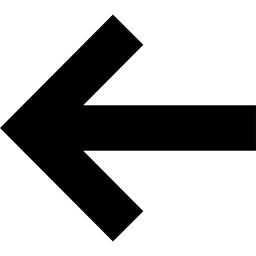# ITensor Basics

The ITensor, or intelligent tensor, is the basic tensor of the ITensor library.

The simplest way to construct an ITensor is to provide its indices:

auto i = Index(2,"index i");
auto j = Index(3,"index j");
auto k = Index(4,"index k");

auto T = ITensor(i,j,k);


This creates an ITensor T with all elements set to zero.

To confirm this is an order 3 tensor (a tensor with 3 indices), call order(T):

println("The order of T is ",order(T));
//prints: The order of T is 3

#include "itensor/all.h"
using namespace itensor;

int main()
{
auto i = Index(2,"index i");
auto j = Index(3,"index j");
auto k = Index(4,"index k");

auto T = ITensor(i,j,k);

println("The order of T is ",order(T));

return 0;
}


### Accessing ITensor Elements

To set a particular element, or component, of an ITensor call its .set method:

T.set(i=2,j=1,k=3, 4.56);


This sets the i=2,j=1,k=3 element of the tensor to the value 4.56.

In a more conventional tensor interface, the above operation might look something like

T[2,1,3] = 4.56; //not actual ITensor code!!


where the user would have to remember that the first entry corresponds to index i, the second to index j, and the third to index k.

For an ITensor, the reason the indices are passed to the .set method along with their values is that nothing about the ITensor interface requires knowing the index order.

If we gave the Index-value pairs such as j=2 in a different order, the .set method still accesses the correct element. A call such as

T.set(k=3,i=2,j=1, 4.56);


has exactly the same outcome as the call

T.set(i=2,j=1,k=3, 4.56);


We can retrieve an element by using the elt function:

auto x = elt(T,k=3,i=2,j=1);
println("x = ",x);
//prints: x = 4.56


We can also set elements of ITensors to be complex numbers:

T.set(i=2,k=3,j=1, 7+8_i);


Now we must use the eltC function to retrieve this element as a complex data type; calling elt would throw an exception:

auto z = eltC(T,i=2,k=3,j=1);
println("z = ",z);
//prints: z = (7,8)


The eltC function always succeeds even if the tensor has only real elements.

### Printing ITensors

A convenient way to print an ITensor is to use the Print macro:

Print(T);
//prints:
// T =
// ITensor ord = 3: (2|id=483|"index i") (3|id=97|"index j") (4|id=922|"index k")


Calling Print(expr) essentially rewrites the code to be println("expr = ",expr), which is why there is a "T = " at the beginning of the output.

The output shows information about the indices, but not the ITensor elements because this could lead to a very large output.

To see the non-zero elements resulting from our earlier calls to .set, we can use the PrintData macro, which prints both the indices and the non-zero elements:

PrintData(T);
//prints:
// T =
// ITensor ord = 3: (2|id=483|"index i") (3|id=97|"index j") (4|id=922|"index k")
// (2,2,1) 7.00+8.00i
// (1,2,3) 4.56+0.00i


### Basic Mathematical Operations

ITensors can be added, subtracted, and multiplied by scalars in the usual way:

auto Q = 2*T;
auto R = Q/3 + T*4_i;
auto S = R - T;
S *= 5;
//etc.


Two ITensors can be added and subtracted if they have the same set of indices, regardless of the index ordering. Internally, the tensor data will be permuted if the index ordering is different, guaranteeing the correct result.

The norm of an ITensor (square root of sum of squared elements) can be computed using the norm function

println("The norm of T is ",norm(T));


For a complete listing of all of the methods of class ITensor, view the detailed documentation.Index ObjectsContracting ITensorsBack to BookBack to Main